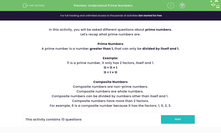# Understand Prime Numbers

In this worksheet, students will be asked to find the difference between prime and composite numbers.Key stage:  KS 2

Curriculum topic:   Number: Multiplication and Division

Curriculum subtopic:   Know Prime Numbers

Popular topics:   Numbers worksheets, Prime Numbers worksheets, Factors worksheets

Difficulty level:#### Worksheet Overview

In this activity, we will be asking different questions about prime numbers.

Let's recap what prime numbers are.

Prime Numbers

A prime number is a number greater than 1, that can only be divided by itself and 1.

Example:

11 is a prime number - it only has two factors, itself and 1.

11 ÷ 11 = 1

11 ÷ 1 = 11

Composite Numbers

Composite numbers are non-prime numbers.

Composite numbers are whole numbers.

Composite numbers can be divided by numbers other than itself and 1.

Composite numbers have more than two factors.

For example, 6 is a composite number because it has the factors: 1, 6, 2, 3.Let's practise identifying different types of numbers in some questions.

### What is EdPlace?

We're your National Curriculum aligned online education content provider helping each child succeed in English, maths and science from year 1 to GCSE. With an EdPlace account you’ll be able to track and measure progress, helping each child achieve their best. We build confidence and attainment by personalising each child’s learning at a level that suits them.

Get started